# 3D Functionals

For sufficiently smooth bodies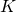, the Minkowski Functionals can be intuitively defined via (weighted) integrals over the volume or boundary of the body.

The scalar functionals can be interpreted as area, perimeter, or the Euler characteristic, which is a topological constant. The vectors are closely related to the centers of mass in either solid or hollow bodies. Accordingly, the second-rank tensors correspond the tensors of inertia, or they can be interpreted as the moment tensors of the distribution of the normals on the boundary.

### Minkowski FunctionalsVolume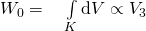Surface area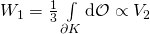Integrated mean curvature
(Mean width for convex bodies)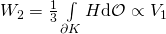Euler Characteristic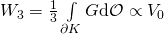with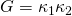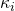= principal curvature

## Cartesian representation (Minkowski Tensors)

Using the position vector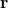and the normal vector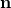on the boundary, the Minkowski Vectors can be defined in the Cartesian representation.

The second-rank Minkowski tensors are defined using the symmetric tensor product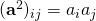.

### Minkowski Vectors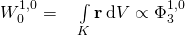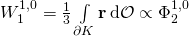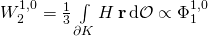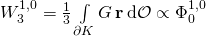### Minkowski Tensors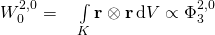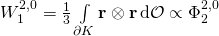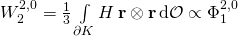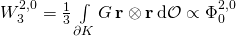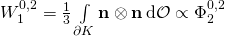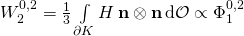Figures are under construction

## Irreducible representation (Spherical Minkowski Tensors)

under construction